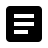## C++ Class and public functions

Before we understand the concept of vptr & vtable, let us recap how we use the class function members. Software component developers design C++ classes with public member functions. These public member functions are used by application developers. Software component developers inherit the base class and override the functions in derived classes. This is how library components are developed. Thus the application calls the derived functions through the base class of the software component. These class design and hierarchy uses compile time linking for calling derived class functions. This design is limited to compiling the application with class libraries. The library is bound in compile time and thus they cannot use dynamic and runtime binding.

## Virtual and pure virtual functions

Practically the compile time design is not used in C++ software components. Software component developers define abstract classes or interface classes. Interface class is nothing but abstract classes i.e. classes with virtual or pure virtual functions. An interface class can be visualized as the C++ equivalent of the API functions used in C. Component developers derives these interface classes and implement the functionalities inside the component software that comes as dynamic link libraries. Here we use virtual and pure virtual functions to call the software classes. The advantage is the functions are not linked in compile time and thus they are not bound during compilation. Here they use dynamic binding or runtime binding or late binding. The compiler uses a hidden pointer member called vptr/vfptr and a table of function pointers.

## C++ vfptr, vftable, virtual functions

C++ compiler creates a hidden class member called virtual-pointer or vfptr in short when there are one or more virtual functions. This vfptr is a pointer that points to a table of function pointers. This table is also created by compiler and called virtual function table or vftable. Each row of the vftable is a function pointer pointing to a corresponding virtual function.

Base object vptr vtable:

 VFPTR VFTABLE FUNCTION vfptr -> base::Vftable -> base::funct1() base::Vftable -> base::funct2()

Derived object vptr vtable:

 VFPTR VFTABLE FUNCTION vfptr -> derived::Vftable -> derived::funct1() derived::Vftable -> derived::funct2()

To accomplish late binding, the compiler creates this vftable table for each class that contains virtual functions and for the class derived from it. The compiler places the addresses of the virtual functions for that particular class in "vftable".

When virtual function call is made through a base-class pointer, the compiler quietly inserts code to fetch the VFPTR and look up the function address in the VFTABLE, thus calling the right function and causing late/dynamic binding to take place.

## Compiler assigns vptr to vtable

class base
{
virtual void funct1(void);
virtual void funct2(void);
};
/* Object construction */
base b;

/* C++ Compiler internally does this*/
b.vtable= &base::funct1;
b.vtable= &base::funct2;

## vfptr, vtables pictorial view

Here is a pictorial view of C++ object with virtual functions. Objects is containing vptr member and how it is pointing to vtable. Further how virtual functions are pointed from vtable entries.## Print vfptr, vtables using C++

Here is a C++ program to print vfptr/vptr and vftable/vtable and function addresses of the vtable.

#include <iostream>
using namespace std;

typedef void (*caller)(void);

class base
{
public:
base()
{
caller vfunc1;
caller vfunc2;
cout << "Object base constructed" <<endl;
cout << "Object address is "<< this <<endl;
cout << "vfptr address is "<< *(void**)this <<endl;
cout << "base::funct1 address is "<< *(void**)(*(void**)this) <<endl;
cout << "base::funct2 address is "<< *((void**)(*(void**)this)+1) <<endl;
cout << "Calling vfunctions using vptr and vtable:" <<endl;

/* Base class vtable function 1, 2 */
vfunc1 = (caller)(*(void**)(*(void**)this));
vfunc2 = (caller)(*((void**)(*(void**)this)+1));

/* Call base class vtable function 1, 2 */
vfunc1();
vfunc2();
}
virtual void funct1(void)
{
cout << "base::funct1" <<endl;
}
virtual void funct2(void)
{
cout << "base::funct2" <<endl;
}
};

class derived : public base
{
public:
derived()
{
caller vfunc1;
caller vfunc2;
cout << "Object derived constructed" <<endl;
cout << "Object address is "<< this <<endl;
cout << "vfptr address is "<< *(void**)this <<endl;
cout << "derived::funct1 address is "<< *(void**)(*(void**)this) <<endl;
cout << "derived::funct2 address is "<< *((void**)(*(void**)this)+1) <<endl;
cout << "Calling vfunctions using vptr and vtable:" <<endl;

/* Derived class vtable function 1, 2 */
vfunc1 = (caller)(*(void**)(*(void**)this));
vfunc2 = (caller)(*((void**)(*(void**)this)+1));

/* Call derived class vtable function 1, 2 */
vfunc1();
vfunc2();
}
void funct1(void);
void funct2(void);
};

void derived::funct1(void)
{
cout << "derived::funct1" <<endl;
}

void derived::funct2(void)
{
cout << "derived::funct2" <<endl;
}

int main (int argh, char *argv[])
{
derived d;
caller vfunc1;
caller vfunc2;
void *** vfptr = (void ***) &d;
void ** vtable = (void **)*vfptr;

/* class vtable function 1, 2 */
vfunc1 = (caller)(vtable);
vfunc2 = (caller)(vtable);
cout << "From main" <<endl;
cout << "Address of d is " << &d << endl;
cout << "d.vfptr is " << vtable << endl;
cout << "Address of d.funct1 is " << vtable << endl;
cout << "Address of d.funct2 is " << vtable << endl;
cout << "Calling vfunctions using vptr and vtable:" <<endl;

/* Call vtable function 1, 2 */
vfunc1();
vfunc2();

return 0;
}

## Print vfptr, vtables using C++ output

The object construction happens in the sequence starting from base class to the derived class. First the base class object is constructed and vptr will point to base::vtable during this time. Later derived class is constructed and this time vptr pointer changes to derived::vtable location. We have taken C style function pointers and assigns these pointers from vtable and vtable. Then we are calling these function addresses using these function pointers.

```Object base constructed
Calling vfunctions using vptr and vtable:
base::funct1
base::funct2
Object derived constructed
Calling vfunctions using vptr and vtable:
derived::funct1
derived::funct2
From main
d.vfptr is 0x104cbe100
Calling vfunctions using vptr and vtable:
derived::funct1
derived::funct2
```

### You may also like

Thanks for reading this answer. We hope you liked the content. These are some relevant contents people also visited. Hope you'll also wish to read these. Understand vptr vtable using C virtual destructor Early binding Late binding virtual base class

You have viewed 1 page out of 62. Your C++ learning is 0.00% complete. Login to check your learning progress.What is the size of a class having one or more virtual functions?
What is the size of a class having one or more virtual functions?How does virtual function work? Understanding vfptr and vftable using C.
Explains how virtual function works. Understanding vfptr and vftable with example using C++ code and same code written in C using pointer and structure.What is early binding and late binding?
What is early binding and late binding?Can a virtual function call from a constructor/destructor work properly?
Can a virtual function call from a constructor/destructor work properly?What is a virtual destructor and its utility?
What is a virtual destructor and its utility?